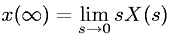Equations > Signal Processing > Laplace Transform Properties > Laplace transform final value theorem (valid if poles of sX(s) are in left half of s plane).

### Laplace transform final value theorem (valid if poles of sX(s) are in left half of s plane).Latex Code:

MathML Code:

 $x\left(\infty \right)=\underset{s\to 0}{lim}\mathrm{sX}\left(s\right)$

MathType 5.0: